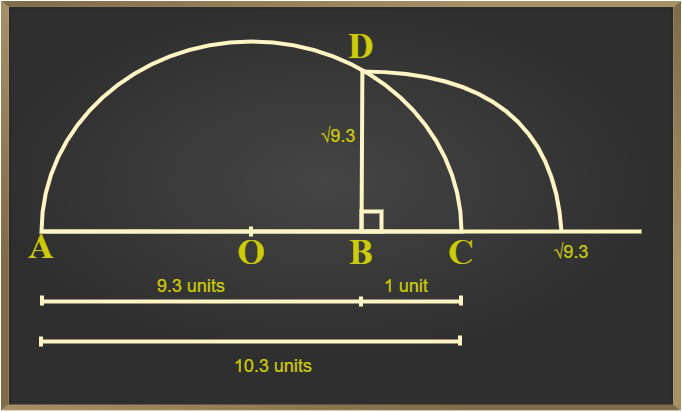Related Articles

# Class 9 NCERT Solutions- Chapter 1 Number System – Exercise 1.5

• Last Updated : 21 Dec, 2020

### Question 1: Classify the following numbers as rational or irrational:

(i) 2 –√5

(ii) (3 +√23)- √23

(iii) 2√7 / 7√7

(iv) 1/√2

(v) 2π

Solution:

(i) 2 –√5

As √5 = 2.2360678… which is non-terminating and non-recurring. It is an irrational number.

When we substitute the value of √5 in equation 2 –√5, we get,

2-√5 = 2-2.2360678…

2-√5 = -0.2360678

Since the number, – 0.2360678…, is a non-terminating and non-recurring,

Therefore, 2 –√5 is an irrational number.

(ii) (3 +√23)- √23

(3 +√23) –√23 = 3+√23–√23

= 3

Since, the number 3 is rational number

Therefore, (3 +√23)- √23 is rational.

(iii) 2√7 / 7√7

2√7 / 7√7 = (2/7)× (√7/√7)

2√7 / 7√7 = (2/7)× (√7/√7)

= (2/7)×1 [As (√7/√7) = 1]

= 2/7

Since the number, 2/7 is in p/q form

Therefore, 2√7/7√7 is rational.

(iv) 1/√2

As, √2 = 1.41421… which is non-terminating and non-recurring. It is a rational number.

When we divide 1/√2 we get,

1/√2 = 1/1.41421…

=0.70710…

Since the number, 0.7071..is a non-terminating and non-recurring,

Therefore, 1/√2 is an irrational number.

(v) 2π

The value of π is 3.1415…

When we substitute the value of π in equation 2π, we get,

2π = 2 × 3.1415…  = 6.2831…

Since the number, 6.2831…, is non-terminating non-recurring,

Therefore, 2π is an irrational number.

### Question 2: Simplify each of the following expressions:

(i) (3+√3)(2+√2)

(ii) (3+√3)(3-√3)

(iii) (√5+√2)2

(iv) (√5-√2)(√5+√2)

Solution:

(i) (3+√3)(2+√2)

After opening the brackets, we get,

(3+√3)(2+√2)= (3×2)+(3×√2)+(√3×2)+(√3×√2)

(3+√3)(2+√2) = 6+3√2+2√3+√6

(ii) (3+√3)(3-√3)

After opening the brackets, we get,

(3+√3)(3-√3) = 32-(√3)2

= 9-3

(3+√3)(3-√3) = 6

(iii) (√5+√2)2

After opening the brackets, we get,

(√5+√2)2 = √52+(2×√5×√2)+ √22       [By using the formula (a + b)2 = a2 + 2ab + b2]

= 5+2×√10+2

(√5+√2)2 = 7+2√10

(iv) (√5-√2)(√5+√2)

After opening the brackets, we get,

(√5-√2)(√5+√2) = (√52-√22

= 5-2

= 3

### Question 3: Recall, π is defined as the ratio of the circumference (say c) of a circle to its diameter, (say d). That is, π =c/d. This seems to contradict the fact that π is irrational. How will you resolve this contradiction?

Solution:

Given π = c/d = 22/7 which is equal to 3.142… which is non-terminating non-recurring decimal.

Therefore, π is irrational.

### Question 4: Represent (√9.3) on the number line.

Solution:

To represent √9.3 on the number line, follow the following steps,

Step 1: Draw a 9.3 units long line segment, name the line as AB.

Step 2: Extend AB to C such that BC=1 unit.

Step 3: Now, AC = 10.3 units. Let the centre of AC be O.

Step 4: Draw a semi-circle with radius OC and centre O.

Step 5: Draw a BD perpendicular to AC at point B which is intersecting the semicircle at D.

Step 6: Join BD.

Step 7: Taking BD as radius and B as the centre point and draw an arc which touches the line segment.

The point where it intersects the line segment is at a distance of √9.3 from B as shown in the figure.### Question 5: Rationalize the denominators of the following:

(i) 1/√7

(ii) 1/(√7-√6)

(iii) 1/(√5+√2)

(iv) 1/(√7-2)

Solution:

(i) 1/√7

Multiply and divide 1/√7 by √7 we get,

(1×√7)/(√7×√7) = √7/7

= √7/7

(ii) 1/(√7-√6)

Multiply and divide 1/(√7-√6) by (√7+√6) we get,

[1/(√7-√6)]×(√7+√6)/(√7+√6) = (√7+√6)/(√7-√6)(√7+√6)

= (√7+√6)/√72-√62 [As, (a+b)(a-b) = a2-b2]

= (√7+√6)/(7-6)

= (√7+√6)/1

= √7+√6

(iii) 1/(√5+√2)

Multiply and divide 1/(√5+√2) by (√5-√2) we get,

[1/(√5+√2)]×(√5-√2)/(√5-√2) = (√5-√2)/(√5+√2)(√5-√2)

= (√5-√2)/(√52-√22) [As, (a+b)(a-b) = a2-b2]

= (√5-√2)/(5-2)

= (√5-√2)/3

(iv) 1/(√7-2)

Multiply and divide 1/(√7-2) by (√7+2) we get,

1/(√7-2)×(√7+2)/(√7+2) = (√7+2)/(√7-2)(√7+2)

= (√7+2)/(√72-22) [As, (a+b)(a-b) = a2-b2]

= (√7+2)/(7-4)

= (√7+2)/3

Attention reader! Don’t stop learning now. Join the First-Step-to-DSA Course for Class 9 to 12 students , specifically designed to introduce data structures and algorithms to the class 9 to 12 students

My Personal Notes arrow_drop_up